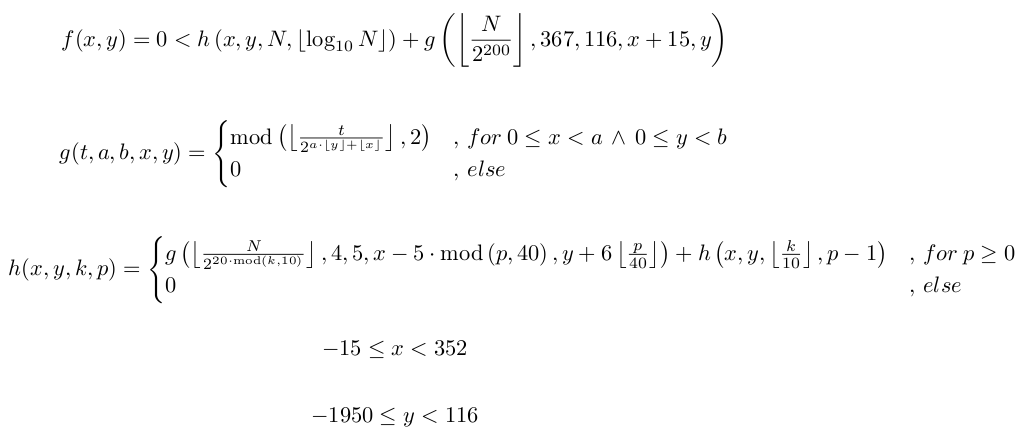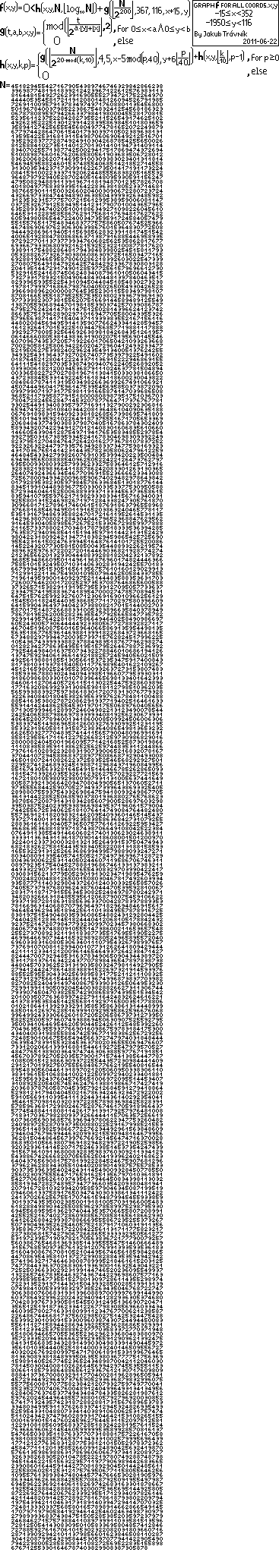## Self Referential Formula in Math

Keywords: math, quine, self-reference, Tupper

Published: 23 Jun 2011 - see updates at the bottom

### Tupper's formula

See newer self-referential stuff: my 2019 self-referential formula uses Bézier curves instead of bitmaps.

I have seen this blog about Tupper self-referential formula recently. This formula is interesting. Its graph contains all possible bitmaps that fit in region of 17 * 106 grid. So it is not much of wonder that one of those many bitmaps contains meaningful representation of the formula itself. Actually, there multiple ways to fit the formula in 17*106 grid, so there are multiple occurrences of the formula in the graph. But there are also all other formulas that fit. It is not truly self-referential since number N which you need to find right viewport is not included inside (see updates at the bottom, Jeff Tupper has produced additional formulas that are truly self-referential, but this famous one is not). You cannot fit a bitmap represented as number in the same bitmap as fonts you would use for base-10 number would take at least 4*3 "pixels" per digit while that digit carries only log210 bits. Is there a better way than that? Some form of compression might work.

### Trávník's formula

So I though how I would design a formula that would be truly self-referential. This design process is more like programming for several reasons:

• I expected it will take one evening (4hrs), but it took three evenings :-)
• I had to debug the formula and refine it until it started to work.
• Formula had to be designed incrementally, in small pieces.
• Getting the picture takes a lot of computational time.

Here is the formula in final form (I will probably describe steps how I arrived to this form in some follow up article if there is enough interest). Originally I have used Haskell in design process. To verify final form, I had to create C program (uses GMP big number library) since Haskell was too slow. This allowed me to verify it again that the formula is matching the program. This is the formula as formated with LyX:Now this one omitted the value of N. The N is very big number, it contains 12876 digits. You can see the N in the graph of the function below. Also, you can copy and paste the N from C source program below. Here is the function as it graphs itself (inside yellow border):You can find source of the program I used to plot it here (it requires GMP big number library to compile and run). At the top of the program there are constants which allow to define viewport you want. It is set to some interesting region that includes part of N. But you can set it to whole region if you have patience. Output graph is printed on terminal, '#' denotes true value, ' ' denotes false values. If you set wider X axis than fits the terminal, you can redirect to file and open in non-word wrapping editor with monospaced font. I have transformed such file to PBM ASCII format and used GIMP to get the image above.

Beware that the C program is very slow. On my computer it ran for about 30 hours. I could optimize it, but I wanted the functions in C to correspond closely to the functions in the formula. While the program uses integer values for x and y computation, you can graph it with real valued x and y too. You can make it significantly faster if you don't want to see all digits of the N in this way. Replace:

```        nnnBase10Len=strlen(strnnn)-1;
```

with

```        nnnBase10Len=60;
```

In this case, you will see only last 60 digits of the N (and they will be shifted to different locations).